# How to convert text to number in Excel

The tutorial shows many different ways to turn a string into a number in Excel: Convert to Number error checking option, formulas, mathematic operations, Paste Special, and more.

Sometimes values in your Excel worksheets look like numbers, but they don't add up, don't multiply and produce errors in formulas. A common reason for this is numbers formatted as text. In many cases Microsoft Excel is smart enough to convert numerical strings imported from other programs to numbers automatically. But sometimes numbers are left formatted as text causing multiple issues in your spreadsheets. This tutorial will teach you how to convert strings to "true" numbers.

## How to identify numbers formatted as text in Excel

Excel has an inbuilt error checking feature that alerts you about possible problems with cell values. This appears as a small green triangle in the top left corner of a cell.  Selecting a cell with an error indicator displays a caution sign with the yellow exclamation point (please see the screenshot below). Put the mouse pointer over the sign, and Excel will inform you about the potential issue: The number in this cell is formatted as text or preceded by an apostrophe.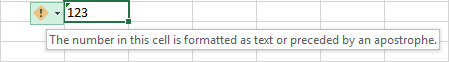In some cases, an error indicator does not show up for numbers formatted as text. But there are other visual indicators of text-numbers:

 Numbers Strings (text values) Right-aligned by default. If several cells are selected, the Status Bar shows Average, Count and SUM. Left-aligned by default. If several cells are selected, the Status Bar only shows Count. The Number Format box displays the Text format (in many cases, but not always). There may be a leading apostrophe visible in the formula bar.

In the image below, you can see the text representations of numbers on the right and actual numbers on the left: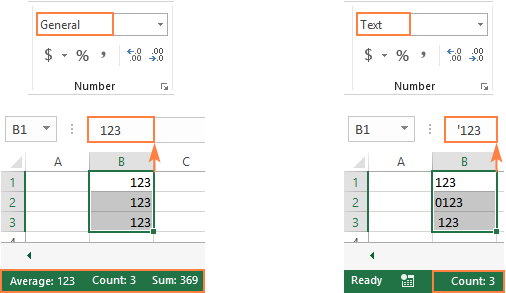## How to convert text to number in Excel

There are a handful of different ways to change text to number of Excel. Below we will cover them all beginning with the fastest and easiest ones. If the easy techniques don't work for you, please don't get disheartened. There is no challenge that cannot be overcome. You will just have to try other ways.

### Convert to number in Excel with error checking

If your cells display an error indicator (green triangle in the top left corner), converting text strings to numbers is a two-click thing:

1. Select all the cells containing numbers formatted as text.
2. Click the warning sign and select Convert to Number.

Done!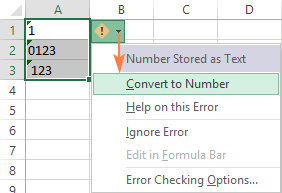### Convert text into number by changing the cell format

Another quick way to convert numerical values formatted as text to numbers is this:

1. Select the cells with text-formatted numbers.
2. On the Home tab, in the Number group, choose General or Number from the Number Format drop-down list.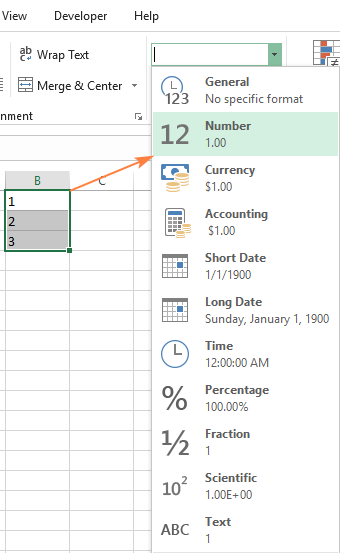Note. This method does not work in some scenarios. For example, if you apply the Text format to a cell, enter a number, and then change the cell format to Number, the cell will remain formatted as text.

### Change text to number with Paste Special

Compared to the previous techniques, this method of converting text to number requires a few more steps, but works almost 100% of time.

To fix numbers formatted as text with Paste Special, here's what you do:

1. Select the text-number cells and set their format to General as explained above.
2. Copy a blank cell. For this, either select a cell and press Ctrl + C or right-click and choose Copy from the context menu.
3. Select the cells you want to convert to numbers, right-click, and then click Paste Special. Alternatively, press the Ctrl + Alt + V shortcut.
4. In the Paste Special dialog box, select Values under in the Paste section and Add in the Operation section.
5. Click OK.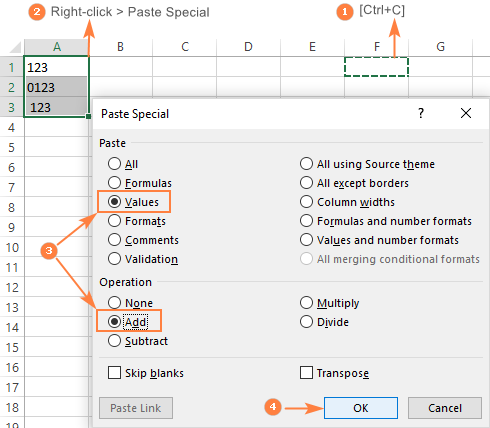If done correctly, your values will change the alignment from left to right, meaning Excel now perceives them as numbers.

### Convert string to number with Text to Columns

It is another formula-free way to convert text to number in Excel. When used for other purposes, for example to split cells, the Text to Columns wizard is a multi-step process. To perform the text to number conversion, you click the Finish button in the very first step :)

1. Select the cells you'd like to convert to numbers, and make sure their format is set to General.
2. Switch to the Data tab, Data Tools group, and click the Text to Columns button.
3. In step 1 of the Convert Text to Columns Wizard, select Delimited under Original data type, and click Finish.

That's all there is to it!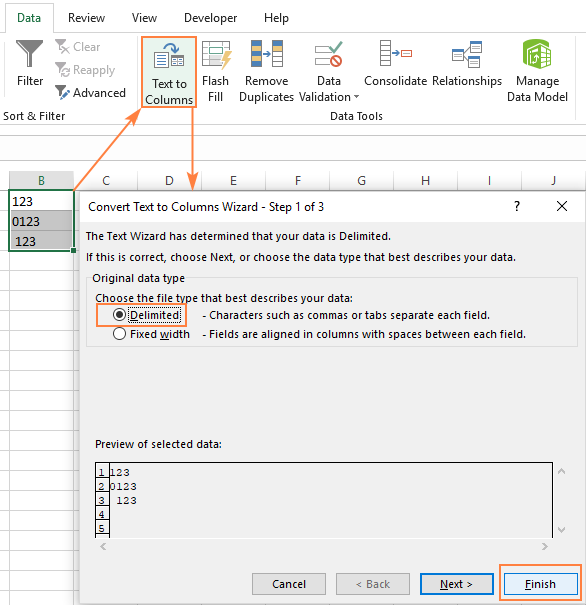### Convert text to number with a formula

So far, we have discussed the built-in features that can be used to change text to number in Excel. In many situations, a conversion can be done even faster by using a formula.

#### Formula 1. Convert string to number in Excel

Microsoft Excel has a special function to convert a string to number - the VALUE function. The function accepts both a text string enclosed in quotation marks and a reference to a cell containing the text to be converted.

The VALUE function can even recognize a number surrounded by some "extra" characters - it's what none of the previous methods can do.

For example, a VALUE formula recognizes a number typed with a currency symbol and a thousand separator:

`=VALUE("\$1,000")`

`=VALUE(A2)`

To convert a column of text values, you enter the formula in the first cell, and drag the fill handle to copy the formula down the column: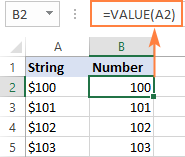#### Formula 2. Convert string to date

Apart from text-numbers, the VALUE function can also convert dates represented by text strings.

For example:

`=VALUE("1-Jan-2018")`

Or

`=VALUE(A2)`

Where A2 contains a text-date.

By default, a VALUE formula returns a serial number representing the date in the internal Excel system. For the result to appear as an actual date, you just have to apply the Date format to the formula cell.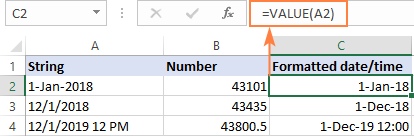The same result can be achieved by using the DATEVALUE function:

`=DATEVALUE(A2)`

#### Formula 3. Extract number from string

The VALUE function also comes in handy when you extract a number from a text string by using one of the Text functions such as LEFT, RIGHT and MID.

For example, to get the last 3 characters from a text string in A2 and return the result as a number, use this formula:

`=VALUE(RIGHT(A2,3))`

The screenshot below shows our convert text to number formula in action: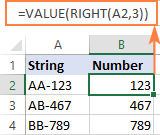If you don't wrap the RIGHT function into VALUE, the result will be returned as text, more precisely a numeric string, which makes any calculations with the extracted values impossible.

### Change Excel string to number with mathematic operations

One more easy way to convert a text value to number in Excel is to perform a simple arithmetic operation that does not actually change the original value. What can that be? For example, adding a zero, multiplying or dividing by 1.

`=A2+0`

`=A2*1`

`=A2/1`

If the original values are formatted as text, Excel may automatically apply the Text format to the results too. You may notice that by the left-aligned numbers in the formula cells. To fix this, be sure to set the General format for the formula cells.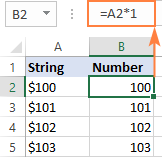Tip. If you'd like to have the results as values, not formulas, use the Paste Special feature to replace formulas with their values.

That's how you convert text to number in Excel with formulas and built-in features. I thank you for reading and hope to see you on our blog next week!

### 47 Responses to "How to convert text to number in Excel"

1.Zeshan Ahmed says:

I want to add text data field in rows portion after Values field in excel pivot table. How can I do that?

2.ANNEE says:

How to change a text into the number like we need to convert "Best" is equal to 5.

•Bob says:

=IF(A1="Best"),5,""). The number 5 may be associated with a certain cell.

3.Migsland says:

You are amazing! Saved my day and future days as well!!

4.Shree says:

Saved my day too..

5.trickyt57 says:

There are a number of tricks you can use if you have a column of numbers in a format that Excel does not recognise as numbers. For example, let's say you have a column of numbers where some idiot has typed each number in using a comma as the thousands separator like this:

52,321.45
17,615.28
19,785.98
41,963.49

If your version of Excel does not use commas for the thousands separator, (mine is et to use the apostrophe - ' -), or if some dummy actually typed in the commas, you can still quickly convert the entire column of text-numbers to numbers by using the Data - Text to columns function:

Highlight the entire column.
Click text to numbers
Select "Delimited" and hit next.
Uncheck all the delimiters and hit next
In the advanced box it asks for the decimals separator and for the thousands separator.
Enter the separator you see on the screen (NOT the separator that you want). So in the above example you would put a comma as the thousands separator, and a full-stop as the decimal separator.
click OK and finish.

Hey presto your text-numbers now ARE numbers in your preferred Excel format. (with an apostrophe as the thousands separator - in my case)

6.Paul says:

Hi

My excel does not recognize the following as a value 2 027,60. I need a thousands separator to be a "," and decimal to be "." . I have a whole column of values that need to be converted.

I have tried the substitute function which works on changing the decimal function for values less than a thousand, thereafter it does not work on a value of 1,000 or more.

Thanks!

7.Vignesh says:

ShortCut key - ALT+a+e+f

•goulag says:

Thanks to you, column to text was causing excel to crash, this was the best solution since i am trying to automate the a script, that does just this.

8.Hubert Soliva says:

Thank you so much to this very helpful post.

9.Waqas says:

Thank you!

10.adam says:

Thank you gurus of excel!

11.Ashwin gajendra says:

1911420211989

12.Ashwin says:

How to convert 1911420211989 this number in word

13.Roger says:

The other problem might be that the 'System Separator' is not the same as your pasted value.
I.e. In the cell you have value 100.22 In other words 100 point 22 or 100 decimal 22.
If your File -> Options -> Advanced -> (About 16 lines down) Use system separator is ticked then the decimal point will make the field text - No matter what you do.
To correct this, un-tick 'Use system separators' and ensure that 'Decimal separator' is a . and 'Thousands separator' is empty and not a blank.

•xyz says:

Thousand separator is taking a , automatically even if we delete it.

14.Ganesh D says:

You guys are making my life easy...
Thanks a lot for your support

15.RAKESH KUMAR JAIN says:

Kindly suggest how to convert all text strings to numbers in a column in Excel sheet? Example:
2,66,020
2,66,020
2,24,650
70,170
91,250
2,66,810
2,66,810
91,250
2,66,810
2,64,840
2,67,720
17,150
2,67,720
2,360
Thanks. RAKESH

•Jay Vyas says:

just replace , with blank and its done :)

16.Myranda says:

I need help making a number in D5 which is 1 to mean 2 in the formula I used in P5.
=(A5/C5)*D5+A5+40

17.RAVI says:

Kindly suggest how to convert all text strings to numbers in a column in Excel sheet? Example:
2,66,020
2,66,020
2,24,650
70,170
91,250
2,66,810
2,66,810
91,250
2,66,810
2,64,840
2,67,720
17,150
2,67,720
2,360

•Aksana (Ablebits.com Team) says:

Hi,
It seems to me that the easiest solution is to apply the ‘Extract’ tool offered by ‘Ablebits Ultimate Suite’. One of the options its menu has is ‘Extract numbers’. Simply click the corresponding radio button on selecting your records, and a new column will appear next to the original one. The extracted data will get numerical status for Excel and lose commas, by the way.

18.Kevin Jernigan says:

I need to give the letter P (for present) the value of 1 and the letter A (for absent) the value of 0. Very new to Excel and can't figure it out. Thank you.

19.Sakandar says:

Hi
How to convert a value number of 3.5581E +14 to the right number. it is not working in with format cells. Thanks for responding.

20.Daan says:

Hi,
I want to convert a placement to an other number, like: placement 1 = 10, placement 2-5 = 7.
What is the best way to do this?

21.Rakesh Kumar says:

How to convert numbers to word.
Exp. 1 - A
2 - B
3 - C

22.guy says:

Finally, Someone who actually knows what they are doing. All of the articles I found including MS only give you one or two options. The Paste Special function is the first time I've seen this method and it did the trick for me. Great article, well organized, comprehensive, and easy to follow. Thank you

23.BH says:

A5 consists of a set of values "3,8,5,6,12" collected from other formulas.
This set of values will have to be used by CHOOSE frequently, CHOOSE(x,A5) where x is a variable.
But, "CHOOSE(x,A5)" returns "#VALUE!".
How can I make it work, please? Thank you very much.

24.Ric Edward Gaspar says:

the value of A1 is "31-35", how can I convert this to (4)? When I use =Value(a1) the result is #value!

25.Gaurav says:

Select text which is to be converted in number

26.Sebplus says:

I have the following challenge, when generating a string that I'm then willing to reference a named variable. Imagine 4 cells in a sheet, such as:
A1 contains the number 9, and I name that cell Blue.Color using Formula/Define Name
A2 contains the number 6, and I name that cell Green.Color
B1 contains the text Green
B2 contains a formula such as =A2&".Color"
The problem is that B2 cell now shows Green.Color, whereas I would have wanted to read 6
Any trick for me ?
Thanks a lot,
Sebplus.

27.sarah guy says:

I want ton convert the following to from the following format
Fri Aug 23 13:05: 39 2019 to dd/mm/yyyy format Is this possible??

28.Gary says:

Thanks Svetlana. Nicely written and organized.

29.Parag says:

So, I have a form created to enter data and the form is linked to excel. The issue I am having is that when someone enters the amount on the form it gets saved as text on Excel. This form is an ongoing process so is there a way to automate the Excel to convert all entries in the Amount column to Number? Instead of converting them everytime manually?

30.AUDRA says:

Can I formulate a word, example Estate to equal 30 days so in the spread sheet when the word estate is used it will calculate 30 days from a specific date in a cell?

31.Joseph Ang says:

How do i change from a qualitative data (Beginning/Developing/Achieving/Exceeding) to a quantitative data (1/2/3/4pts) when a person key in this in google excel:
Beginning = 1pt
Developing = 2pts
Achieving = 3pts
Exceeding = 4pts

32.Wasi says:

'0200009782563
0200009782563
Both cell len show 13. How I found out both cell data or len different?

33.Steph says:

Is there a way to do the following: I have a row and in it, it has Single and Family mixed all the way through. I want it to basically calculate how many Single cells there are but a single cell is a certain cost like \$50. The same with Family, how many family but the price for family is \$100. So it won't actually tell me there are 4 single and 8 family, it would give me the total price tag.

34.Georgina says:

In order to use Regression in the data analysis I need to have numeric data. However some of it is blank using "" in a previous formula, but this is recognised as text. Do you have any suggestions as to what I can put instead of "" which represents blank but is not text?

NULL would also be text of course...

35.Donna says:

How to create a sum field that totals columns that were converted to numeric via Data >Tools> Text to Columns. Sum function not recognizing values in columns converted as numeric, even with format changed to numeric.

36.Keshav says:

I want to change the text into number by following way:
abc = (1+2+3) = 6
bcd = (2+3+4) = 9
cde = (3+4+5) = 12

Pls Help. Thank You in Advance.

37.Marcin says:

I have pasted a large number of values which have "." as a separator (example 23.310
). I need to convert this to either "," (example 23,310) or no separator at all. I have tried formatting the cell to currency, general and few other just to get a value to do calculations and it doesn't work. Is there a command which would replace all "." to ","?

•Ricardo says:

Hi Marcin.
Same problem here. Some logs have 23.310 instead of 23,310 and import them to excel doesn't change the format.

A very quick solution that worked for me:
- Choose the column with mouse
- Use replace function -> replace . for , -> it's text so it's works with no problem
- If necessary format the column to number after it replacing

38.Paulo says:

I want to separate this number in 2 columns, breaking it where the comma is...

"25,10"

The result I want to obtain is 25 and 10. But when I convert it I get 25 and 1.
what am I doing wrong?

Best,
PM

39.Jack says:

How do you convert a text of .01- to a number -.01?

•jack says:

Nevermind i figured it out, sorry to bother you

40.Ibukun says:

your tip really helped me on how to change text number to number, as I have been on it for days, i initially thought my excel wasn't working. This has been helpful. Thanks

60+ professional tools for Excel